# An introduction to CNNs and a step by step model of a Digit Recognizer using MNIST database in…

Convolutional Neural Networks (CNNs or ConvNets) were introduced towards the end of 20th century but did not gain popularity due to high computational cost. CNNs came into limelight after AlexNet won the ImageNet challenge in 2012. Now, with an increased availability of GPUs and TPUs, ConvNets are being widely used. This article will introduce the basic outline of a CNN which will be followed by a complete solution of the MNIST database (Handwritten Digit Recognition).

### Overview

The topics that will be covered to understand the basic structure and working of ConvNets are :

1. Convolution operation (For edge detection) : Working of a filter, Padding and Striding.
2. Convolutions over Volume
3. Building a CNN
4. Pooling Layer
5. Fully Connected Layer

### Convolution operation for edge detection

We use filters (or kernels) for edge detection. The filters can be horizontal, vertical or even tilted at an angle such as 45 degrees.The working of a vertical detector is shown below: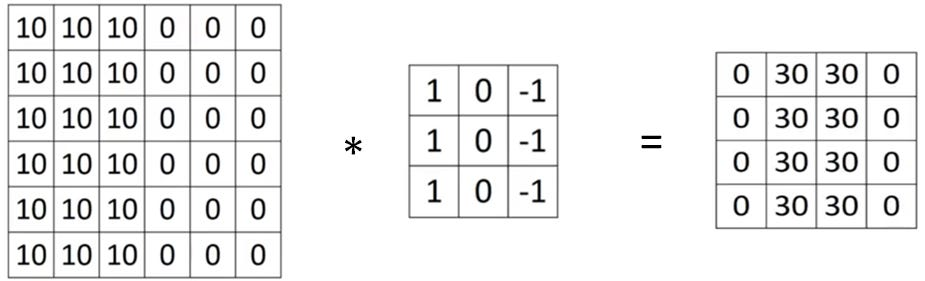The input matrix (6x6x1) convolves with the filter matrix(3x3x1) to give the output matrix(4x4x1)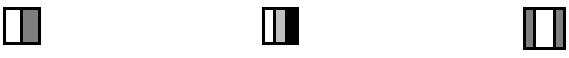We notice an edge in the output matrix(white color) which gets thinner as the size is increased

The filter matrix scans the input image 9(3×3) elements at a time starting from the top left corner. Element by element multiplication is followed by addition of all the nine products to form one element of the output matrix.

First iteration : Filter scans columns 1 to 3 and rows 1 to 3.

Second iteration : Filter scans columns 2 to 4 and rows 1 to 3 and so on.

Last iteration : Filter scans columns 4 to 6 and rows 4 to 6.

Thus, the output image has the size (6–3 +1) x (6–3+1).

If the input matrix has nxn elements and the filter has fxf elements, the output matrix has (n-f+1) x (n-f +1) elements.

According to mathematics, this process is called cross-correlation but it is named convolution by convention in Neural Networks.

#### Padding

The process of convolution has drawbacks as the size of the image reduces. Also the pixels in the corners are counted only once as there is no overlapping which leads to loss of information around the corners. Padding helps in overcoming these disadvantages.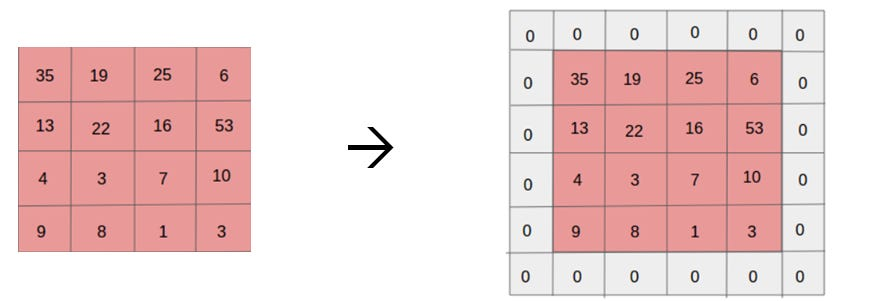A 4×4 image padded with a single layer of zeros (p=1)

Now, if the input matrix has nxn elements, p is the padding and the filter has fxf elements, the output matrix has (n+2p-f+1) x (n+2p-f +1) elements. This helps us give more importance to the pixels in the corners.

#### Striding

The filter matrix scans the input matrix 9 elements at a time in a continuous manner as explained before. The stride(s) is set to 1 by default. If we want to skip one iteration after every iteration, we can set strides = 2. The number of iterations is reduced in a regular manner. This can be useful when the size of the image is large. If the stride gives a circumstance where some part of the filter doesn’t completely overlap the input matrix, we do not consider it.

Thus, with the combined effect of padding and striding in convolution :

The value of the output above is not always an integer. The cases where the value is not an integer, we take the floor value.

#### Convolutions over Volume

1. With a single filter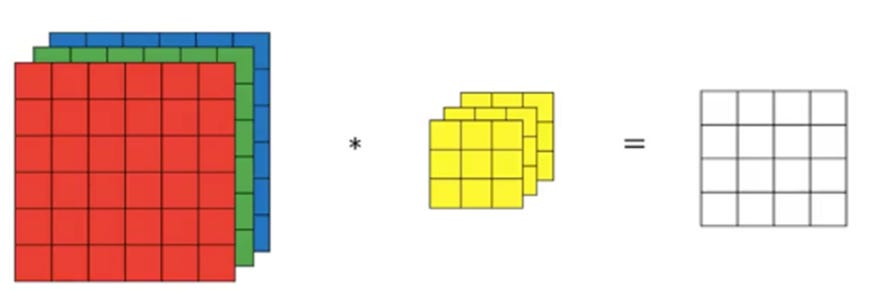6x6x3 input matrix convolves with 3x3x3 filter to give 4x4x1 output

The input matrix has the dimensions n(h) x n(w) x n(c) [height, width and no of channels]. The number of channels should be same for the input matrix and the filter.The values of n(h) and n(w) can be different. In this example, the filter can be thought of as a cube which moves over 27 elements of the input at a time starting from the top left corner. Thus, no matter what the number of channels is, input with one filter will always give a single channeled output.

2.Using more than one filters (multiple filters)

The number of channels in the input and the filter will still be the same. The no of channels of the output will be equal to the number of filters used.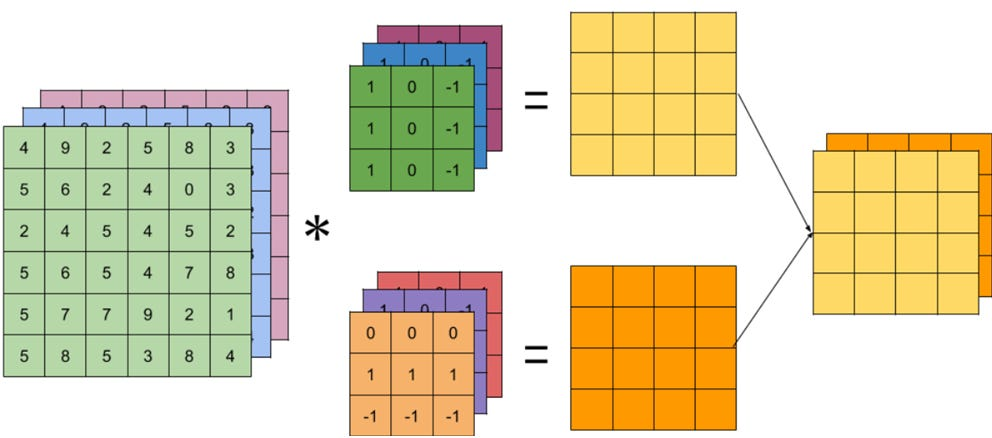The number of channels in the output layer is equal to the number of filters

#### Building a CNN

Now that we have understood the concept of convolution, I will try to justify the reason these are considered to be a class of Neural Networks. The elements of the filter (9 in a 3×3 filter) can be trained in a fashion similar to ANNs. We can also add a bias term and a non linear activation function. For a single 3×3 filter we have 10 parameters (9 weights and 1 bias term). This computation from input layer to the output layer using one(or multiple) filter is called one layer of a CNN. We can add many such layers in our network. The advantage of CNNs over traditional NNs lies in the fact that CNNs are less prone to overfitting as the number of parameters is independent of the size of the input.

For a single layer of a CNN :

1. Input : n(h) x n(w) x n(c)
2. Filter(s) : f x f x n(c) and number of filters is n(c)’
3. Output :

4. Number of weights : [n(h) x n(w) + 1] x n(c)’

### Pooling Layer

ConvNets use pooling layers to reduce the size of representation and increase the speed of computation. An important fact about the pooling layer is that there are no parameters. We only give a set of hyperparameters (size of filter(f), strides(s) and type(max or avg)) for the pooling layer. As there will be no parameters to learn during back propagation, we can say that pooling is like a fixed function. The convolution layer and pooling layer are usually considered as a same layer as pooling layer does not have any parameters.

The most common types of pooling are:

1. Max pooling : The filter defined as a hyperparameter will select the maximum value from the set of elements under concidertion. Max pooling is more widely used than average pooling.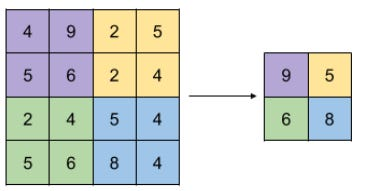Max Pooling

2. Average Pooling : The filter defined as a hyperparameter will select the average value from the set of elements under concidertion. This is used in very deep NNs.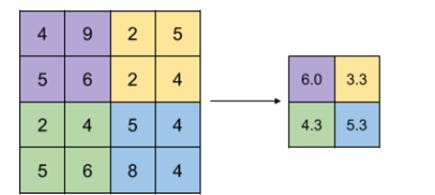Avg Pooling

### Fully Connected Layer

The fully connected layer is just like a single NN layer. The output of the previous layer is given as an input to this layer. This layer gets its name from the fact that each unit of the previous layer is connected to each unit in this layer.

### PART — B(Step by step solution of MNIST database)

Our goal is to correctly identify digits from a dataset of tens of thousands of handwritten images. The data can be found at : https://www.kaggle.com/c/digit-recognizer/data

Steps towards completion of our first CNN project :

1.We first import the libraries required.

`import pandas as pdimport numpy as npimport matplotlib.pyplot as pltimport matplotlib.image as mpimgimport seaborn as sns`
`from sklearn.model_selection import train_test_split`
`from keras.utils.np_utils import to_categorical from keras.models import Sequentialfrom keras.layers import Dense, Dropout, Flatten, Conv2D, MaxPool2Dfrom keras.optimizers import RMSpropfrom keras.preprocessing.image import ImageDataGeneratorfrom keras.callbacks import ReduceLROnPlateau`

2.We load the files and assign the labels to Y_train. These are one hot encoded and the remaining data is given to X_train’

`train = pd.read_csv("../input/train.csv")test = pd.read_csv("../input/test.csv")Y_train = train["label"]X_train = train.drop(labels = ["label"],axis = 1) Y_train = to_categorical(Y_train, num_classes = 10)`

3.We check for null values. There are no null values in the dataset.

`X_train.isnull().any().describe()test.isnull().any().describe()`

4.Dividing by 255 is a simple and efficient way for normalization of pixels. CNNs converge faster on [0,1] data than on [0,255].

`X_train = X_train / 255test = test / 255`

5.We use reshape as the input values are in 1-D but we want a 2-D form for n(h) x n(w). Then we split the training set into 10% validation and 90% training data.

`X_train = X_train.values.reshape(-1,28,28,1)test = test.values.reshape(-1,28,28,1)X_train, X_val, Y_train, Y_val = train_test_split(X_train, Y_train, test_size = 0.1, random_state=2)`

6.These are the first 4 layers of our model. The Conv2D layers followed by max pool layers are considered to be a single layer. Dropout is a regularization technique for reducing overfitting. It refers to dropping out units (both hidden and visible) in a neural network.

`model = Sequential()`
`model.add(Conv2D(filters = 32, kernel_size = (5,5),padding = 'Same',                  activation ='relu', input_shape = (28,28,1)))model.add(Conv2D(filters = 32, kernel_size = (5,5),padding = 'Same',                  activation ='relu'))model.add(MaxPool2D(pool_size=(2,2)))model.add(Dropout(0.25))model.add(Conv2D(filters = 64, kernel_size = (3,3),padding = 'Same',                  activation ='relu'))model.add(Conv2D(filters = 64, kernel_size = (3,3),padding = 'Same',                  activation ='relu'))model.add(MaxPool2D(pool_size=(2,2), strides=(2,2)))`

7.These are fully connected layers .We add softmax activation unit in the end for a softmax output. Flatten is used to convert the 2-D output back to 1-D as it has to enter a fully connected layer.

`model.add(Dropout(0.25))model.add(Flatten())model.add(Dense(256, activation = "relu"))model.add(Dropout(0.5))model.add(Dense(10, activation = "softmax"))`

8.We set RMSprop as our optimizer. The learning rate will become half its value if accuracy remains unchanged for 3 iterations.

`optimizer = RMSprop(lr=0.001, rho=0.9, epsilon=1e-08, decay=0.0)model.compile(optimizer = optimizer , loss = "categorical_crossentropy", metrics=["accuracy"])epochs = 30batch_size = 64learning_rate_reduction = ReduceLROnPlateau(monitor='val_acc',                                             patience=3,                                             verbose=1,                                             factor=0.5,                                             min_lr=0.00001)`

9.Finally, we fit our model. This is the stage where the forward and backward propagation occur. This step will take around 2 hours for computation on a CPU if the number of epochs are 30.We finally predict our test labels.

`final = model.fit(X_train, Y_train, batch_size = batch_size, epochs = epochs, validation_data = (X_val, Y_val),callbacks=[learning_rate_reduction])results = model.predict(test)`

https://www.kaggle.com/mridul02/digit-recognizer-cnn-keras is the link to my kaggle kernel on Digit Recognizer. The accuracy of the test set is 99.58%.

To get a better insight on the capability of CNNs, try to apply other machine learning algorithms and compare the results.

### Conclusions

In this article, we have understood the working of a ConvNet and implemented it on a famous machine learning problem. After completing the article, we know :

1. The working of ConvNets and why they are called so.
2. Why CNNs work better than traditional ANNs.
3. How to build a basic CNN model.

I have put in all my knowledge and effort into making this as informative and compact as possible. I welcome feedbacks and would appreciate an advice on how to make this article better.

Source: Deep Learning on Medium